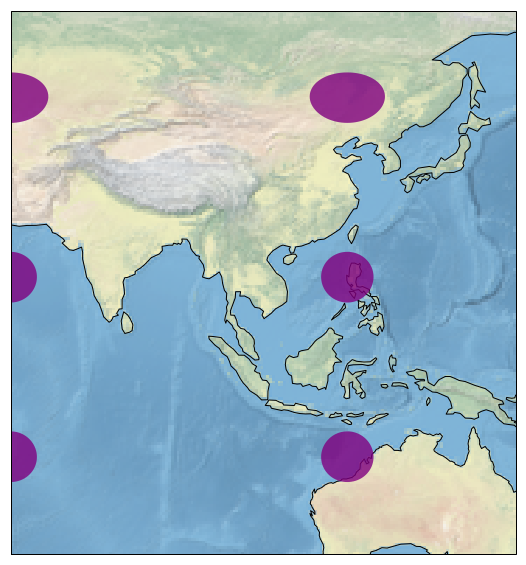# (32)Python时间序列

``````import matplotlib.pyplot as plt
import cartopy.crs as ccrs

fig = plt.figure(figsize=(15, 10))
ax = fig.add_subplot(1, 1, 1, projection=ccrs.PlateCarree())

# make the map global rather than have it zoom in to
# the extents of any plotted data

ax.set_extent((60, 150, 55, -25))

ax.stock_img()
ax.coastlines()

ax.tissot(facecolor='purple', alpha=0.8)

plt.show()
```Python```关注右侧公众号，随时随地查看教程
Python数据分析教程目录